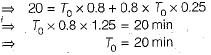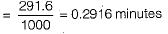Courses

# Test: Work Study - 2

## 10 Questions MCQ Test Topicwise Question Bank for Mechanical Engineering | Test: Work Study - 2

Description
This mock test of Test: Work Study - 2 for Mechanical Engineering helps you for every Mechanical Engineering entrance exam. This contains 10 Multiple Choice Questions for Mechanical Engineering Test: Work Study - 2 (mcq) to study with solutions a complete question bank. The solved questions answers in this Test: Work Study - 2 quiz give you a good mix of easy questions and tough questions. Mechanical Engineering students definitely take this Test: Work Study - 2 exercise for a better result in the exam. You can find other Test: Work Study - 2 extra questions, long questions & short questions for Mechanical Engineering on EduRev as well by searching above.
QUESTION: 1

Solution:
QUESTION: 2

Solution:
QUESTION: 3

### Standard time is obtained from normal time by adding the policy allowance and

Solution:
QUESTION: 4

The standard time for an operation has been calculated as 20 min. The worker was rated at 80%. If the relaxation and other allowances were 25%, then the observed time would be

Solution:

Standard time = Normal time + Allowances
= (observed time x rating factor) + allowancesQUESTION: 5

Which one of the following chart gives simultaneous information about the progress of work and machine loading:

Solution:
QUESTION: 6

A diagram showing the path followed by men and materials while performing a task is known as

Solution:
QUESTION: 7

Consider the following work sampling data: Working time = 60%, average rating = 90%, relaxation allowance = 12.5% actual output during the study = 1000 units and study duration = 480 minutes. The standard time per unit (in minutes) will be

Solution:

Observed time = 480 x 0.6
= 288 mins.
Normal time = Observed time x rating  factor
= 288 x 0.9
= 259.2 mins.
Standard time = Normal time x allowances
= 259.2 x 1.125
= 291.6 minutes
∴ The standard time per unitQUESTION: 8

Merit rating is the method of determining

Solution:
QUESTION: 9

Job evaluation is the method of determining

Solution:
QUESTION: 10

What term is used for the procedure of modifying work constant where by more meaning and enjoyment is given to the job by involving employees in planning, organization and control of their work?

Solution: